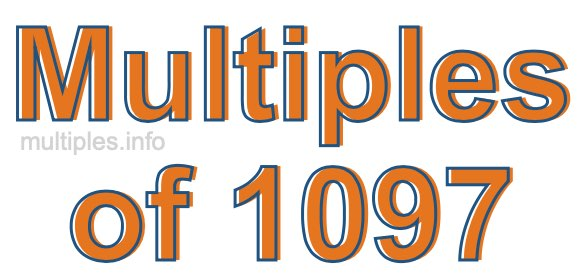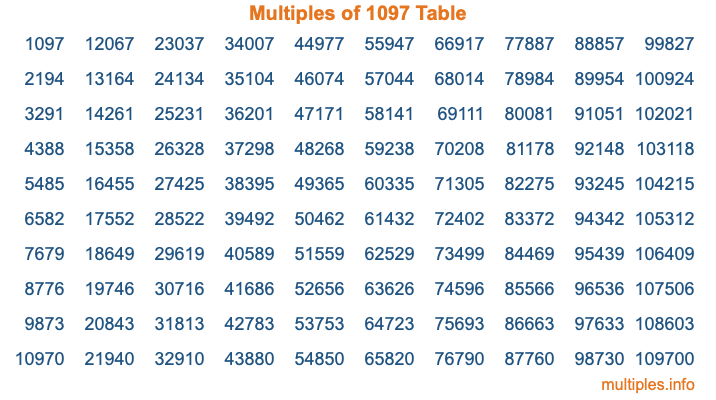Multiples of 1097Welcome to the Multiples of 1097 page. Here we will first teach you everything you will ever need to know about the multiples of 1097, and then give you a study guide summary of everything we taught you to make sure you remember it all. Use this page to look up facts and learn information about the multiples of 1097. This page will make you a multiples of one thousand ninety-seven expert!

Definition of Multiples of 1097
Multiples of 1097 are all the numbers that when divided by 1097 equal an integer. Each of the multiples of 1097 are called a multiple. A multiple of 1097 is created by multiplying 1097 by an integer.

Therefore, to create a list of multiples of 1097, you start with 1 multiplied by 1097, then 2 multiplied by 1097, then 3 multiplied by 1097, and so on for as long as you want. Thus, the list of the first five multiples of 1097 is 1097, 2194, 3291, 4388, and 5485. To see a larger list of multiples of 1097, see the printable image of Multiples of 1097 further down on this page. We also have a category where you can choose any nth multiple of 1097.

Multiples of 1097 Checker
The Multiples of 1097 Checker below checks to see if any number of your choice is a multiple of 1097. In other words, it checks to see if there is any number (integer) that when multiplied by 1097 will equal your number. To do that, we divide your number by 1097. If the the quotient is an integer, then your number is a multiple of 1097.

Is  a multiple of 1097?

Least Common Multiple of 1097 and ...
A Least Common Multiple (LCM) is the lowest multiple that two or more numbers have in common. This is also called the smallest common multiple or lowest common multiple and is useful to know when you are adding our subtracting fractions. Enter one or more numbers below (1097 is already entered) to find the LCM.

Check out our LCM Calculator if you need more details about the Least Common Multiple or if you need the LCM for different numbers for adding and subtraction fractions.

nth Multiple of 1097
As we stated above, 1097 is the first multiple of 1097, 2194 is the second multiple of 1097, 3291 is the third multiple of 1097, and so on. Enter a number below to find the nth multiple of 1097.

th multiple of 1097

Multiples of 1097 vs Factors of 1097
1097 is a multiple of 1097 and a factor of 1097, but that is where the similarities end. All postive multiples of 1097 are 1097 or greater than 1097. All positive factors of 1097 are 1097 or less than 1097.

Below is the beginning list of multiples of 1097 and the factors of 1097 so you can compare:

Multiples of 1097: 1097, 2194, 3291, 4388, 5485, etc.

Factors of 1097: 1, 1097

As you can see, the multiples of 1097 are all the numbers that you can divide by 1097 to get a whole number. The factors of 1097, on the other hand, are all the whole numbers that you can multiply by another whole number to get 1097.

It's also interesting to note that if a number (x) is a factor of 1097, then 1097 will also be a multiple of that number (x).

Multiples of 1097 vs Divisors of 1097
The divisors of 1097 are all the integers that 1097 can be divided by evenly. Below is a list of the divisors of 1097.

Divisors of 1097: 1, 1097

The interesting thing to note here is that if you take any multiple of 1097 and divide it by a divisor of 1097, you will see that the quotient is an integer.

Multiples of 1097 Table
Below is an image of the first 100 multiples of 1097 in a table. The table is in chronological order, column by column. The first column has the first ten multiples of 1097, the second column has the next ten multiples of 1097, and so on.The Multiples of 1097 Table is also referred to as the 1097 Times Table or Times Table of 1097. You are welcome to print out our table for your studies.

Negative Multiples of 1097
Although not often discussed or needed in math, it is worth mentioning that you can make a list of negative multiples of 1097 by multiplying 1097 by -1, then by -2, then by -3, and so on, to get the following list of negative multiples of 1097:

-1097, -2194, -3291, -4388, -5485, etc.

Multiples of 1097 Summary
Below is a summary of important Multiples of 1097 facts that we have discussed on this page. To retain the knowledge on this page, we recommend that you read through the summary and explain to yourself or a study partner why they hold true.

There are an infinite number of multiples of 1097.

A multiple of 1097 divided by 1097 will equal a whole number.

1097 divided by a factor of 1097 equals a divisor of 1097.

The nth multiple of 1097 is n times 1097.

The largest factor of 1097 is equal to the first positive multiple of 1097.

1097 is a multiple of every factor of 1097.

1097 is a multiple of 1097.

A multiple of 1097 divided by a divisor of 1097 equals an integer.

1097 divided by a divisor of 1097 equals a factor of 1097.

Any integer times 1097 will equal a multiple of 1097.

Multiples of a Number
Here you can get the multiples of another number, all with the same attention to detail as we did for multiples of 1097 on this page.

Multiples of
Multiples of 1098
Did you find our page about multiples of one thousand ninety-seven educational? Do you want more knowledge? Check out the multiples of the next number on our list!